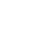×Want to shop in US Dollar? Try our Webshop specifically for North America!

# Rammstein Boots

•••••100.00 EUR
In stock.

10 hole "RAMMSTEIN" leather boots with logo embroidery on the outer side, with stable screwed, sewn and glued rubber sole and steelcaps in the toes of the boots.

Material: 100% leather, rubber sole.

Bootleg height: 18 cm.

Please do NOT use the box as a shipping box for returns.

UK - EU - US

3 = 36 = 5
3.5 = 37 = 5.5

4 = 38 = 6
5 = 39 = 7
6 = 40 =7.5
7 = 41 = 8.5
8 = 42 = 9
9 = 43 =10
10 = 44 = 10.5
11 = 45 = 11.5
12 = 46 = 12
13 = 47 = 13